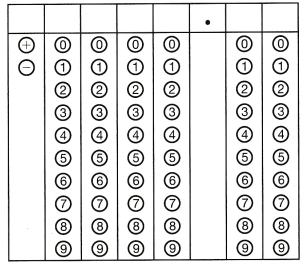Refer to our Texas Go Math Grade 6 Answer Key Pdf to score good marks in the exams. Test yourself by practicing the problems from Texas Go Math Grade 6 Unit 1 Study Guide Review Answer Key.

Question 1.
Graph each number on the number line.
7, – 2, 5, 1, – 1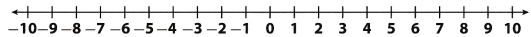Write the opposite of each number.

Question 2.
8 ___________
The opposite of a number is the number on the other side of the 0 number line, and the same distance from 0.
Opposite of 8 is – 8

Go Math 6th Grade Math Unit 1 Study Guide Answer Key Question 3.
– 3 ___________
The opposite of a number is the number on the other side of 0 on the number tine and the same distance from 0.
Opposite of – 3 is 3.

List the numbers from the greatest of each number.

Question 4.
4, 0, – 2, 3 ___________
Number – 2 is less than 0 which is less than 3 which is less than 4.
Therefore, we list them as: – 2, 0, 3, 4

Question 5.
– 3, – 5, 2, – 2 ___________
Number – 5 is less than – 3 which is less than – 2 which is less than 2
Therefore, we list them as: – 5, – 3, – 2, 2

Use a number line to help you compare the numbers. Use < or >.

Question 6.
4 _________ 1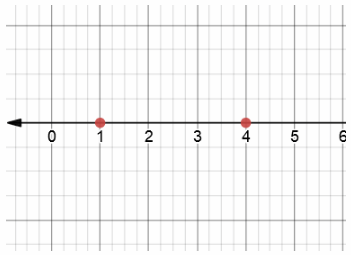It is clear that 1 is less than 4.
So, we can write: 1 < 4

Question 7.
– 2 _________ 2It is clear that – 2 is less than 2.
So, we can write: – 2 < 2

Question 8.
– 3 _________ – 5It is clear that – 3 is less than – 5.
So, we can write: – 3 > – 5

Unit 1 Study Guide Answer Key Go Math Grade 6 Question 9.
– 7 _________ 2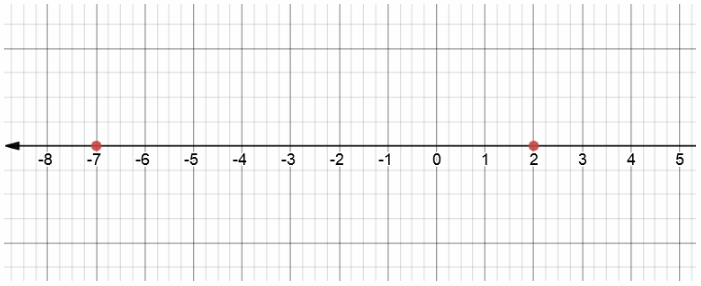It is clear that – 7 is less than 2.
So, we can write: – 7 < 2

Find each absolute value.

Question 10.
|6| _________
Absolute value of some number is its distance from 0 on the number line!
Therefore, we know:
|6| = 6

Question 11.
|- 2| _________
Absolute value of some number is its distance from 0 on the number line!
Therefore, we know
|- 2| = 2

Classify each number by indicating in which set or sets it belongs.

Question 1.
8
Number 8 is positive Whole number.
Therefore, it belongs to set of Whole numbers, Integers and Rational numbers!

Question 2.
0.25
Number 0.25 is Rational number.
Therefore, it belongs to set of Rational numbers!

Find the absolute value of each rational number.

Question 3.
|3.7| _______________
Absolute value of some number is its distance from 0 on the number line!
Therefore, we know
|3.7| = 3.7

Question 4.
|- $$\frac{2}{3}$$|
Absolute value of some number is its distance from 0 on the number line!
Therefore, we know
|- $$\frac{2}{3}$$| = $$\frac{2}{3}$$

Graph each set of numbers on the number line and order the numbers from greatest to least.

Question 5.
– 0.5, – 1, –$$\frac{1}{4}$$, 0Firstly, we transform fractions into decimals!
–$$\frac{1}{4}$$ = – 0.25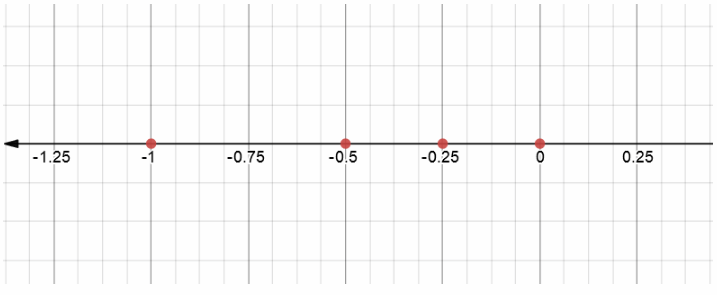Now we can say that:
Number 0 is greater than – 0.25 which is greater than – 0.5 which is greater than – 1.

Division: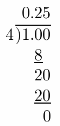Question 1.
CAPEEPS IN MATH Climatologist Each year a tree is alive, it adds a layer of growth, called a tree ring, between its core and its bark. A climatologist measures the width of tree rings of a particular tree for different years: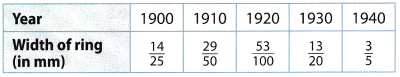The average temperature during the growing season is directly related to the width of the ring, with a greater width corresponding to a higher average temperature.

a. List the years in order of increasing ring width.
Here. we want to order years based on va1ue given below, from least increase to greatest increase!
Firstly, we get all fractions to have common denominator which, in this case, will be 100.Now, we compare numerators 53, 58, 56, 60, 65
Number 53 is less than 56 which is less than 58 which is less than 60 which is less than 65.
So, we can write them us:
35 < 56 < 58 < 60 < 65
Final thing to do ¡s to return numbers into given formats and write solution
$$\frac{53}{100}<\frac{14}{25}<\frac{29}{50}<\frac{3}{5}<\frac{13}{20}$$
Now, we want to write years corresponding to width of ring in increasing order:
1920, 1900, 1910, 1940, 1930

b. Which year was hottest? How do you know?
Hottest year, by previous calculations was 1930
we can say that because we know that 1930, the width of ring was greatest!

c. Which year was coldest? How do you know?
Coldest year, by previous calculations, was 1920
We can say that because we know that in 1920, the width of the ring was the least

Multiplication: You can do it by yourselves as an exercise!

A parking garage has floors above and below ground level. For a scavenger hunt, Gaia’s friends are given a list of objects they need to find on the third and fourth level below ground, the first and fourth levels above ground, and ground level.

a. If ground level is 0 and the first level above ground is 1, which integers can you use to represent the other levels where objects are hidden? Explain your reasoning.
As we know that the ground level is labeled as 0 and that the first level above ground is labeled as 1, we can conclude that the third level below ground is labeled as – 3,
fourth level below ground is Labeled as – 4 and the fourth level above ground is labeled as 4.
Our reasoning is quite simple here, we present levels below ground as negative integers, and levels above ground as positive integers

b. Graph the set of numbers on the number line.c. Gala wants to start at the lowest level and work her way up. List the levels in the order that Gala will search them.
As we can see on the number line above, Gaia will have to search levels in order.
– 4, – 3, 0, 1, 4
Note that reading our number line from left to right allows us to order numbers from least to greatest.

d. If she takes the stairs, how many flights of stairs will she have to climb? How do you know?
As we can see on the number line above, lowest level is 4
If she take the stairs, she will have to pass some levels that she won’t search.
If she starts at lowest level – 4, then she will have to climb 4 flights of stairs in order to get to ground Level 0.
Also, after she reaches 0 level she still has 4 flights of stairs to climb till fourth level above ground!
ALtogether, she has to climb:
4 + 4 = 8 flights of stairs!
We can also observe this using absolute value, as we are speaking about distance, 4 levels means 4 flights of stairs, so it doesn’t matter whether we are above/below ground, we are crossing same distance.

Selected Response

Question 1.
What is the opposite of – 9?
(A) 9
(B) –$$\frac{1}{9}$$
(C) 0
(D) $$\frac{1}{9}$$
(A) 9

Explaination:
The opposite of a number ¡s the number on the other side of 0 number line, and the same distance from 0.
Opposite of – 9 is 9.

Question 2.
Kyle is currently 60 feet above sea level. Which correctly describes the opposite of Kyle’s elevation?
(A) 60 feet below sea level
(B) 60 feet above sea level
(C) 6 feet below sea level
(D) At sea level
(A) 60 feet below sea level

Explaination:
The opposite of a number is the number on the other side of 0 number tine, and the same distance from 0. We can use this statement in our case!
As kyle is 60 feet above sea level, opposite of his elevation would be: 60 feet below sea level!

What is the absolute value of 27?
(A) – 27
(B) 0
(C) 3
(D) 27
(D) 27

Explaination:
Absolute value of some number is its distance from 0 in the number line.
|27| = 27

Question 4.
In Albany it is – 4°F, in Chicago it is – 14°F, in Minneapolis it is – 11°F, and in Toronto it is – 13°F. In which city is it the coldest?
(A) Albany
(B) Chicago
(C) Minneapolis
(D) Toronto
(B) Chicago

Explaination:
Here, we will compare given temperature to find out, which is lowest.
When we find lowest temperature, we will know which city is the oldest!

Temperature of – 4°F is greater than – 1°F which is greater than – 13°F which is greater than – 14°F.
Now, we can concLude that the lowest temperature is -14°F.
That temperature is measured in Chicago.
So, coldest is in Chicago.

Question 5.
Which shows the integers in order from greatest to least?
(A) 18, 4, 3, – 2, – 15
(B) – 2, 3, 4, – 15, 18
(C) – 15, – 2, 3, 4, 18
(D) 18, – 15, 4, 3, 2
(A) 18, 4, 3, – 2, – 15

Explaination:
We have integers 18, 4, 3, – 2, – 15 given!
We want to order them from greatest to least.

Number 18 is greater than 4 which is greater than 3 which is greater than – 2 which is greater than – 15.
Therefore, we can write:
18 > 4 > 3 > – 2 > – 15
Order from greatest to least is: 18, 4, 3, – 2, – 15.

Question 6.
Joanna split three pitchers of water equally among her eight plants. What fraction of a pitcher did each plant get?
(A) $$\frac{1}{8}$$ of a pitcher
(B) $$\frac{1}{3}$$ of a pitcher
(C) $$\frac{3}{8}$$ of a pitcher
(D) $$\frac{8}{3}$$ of a pitcher
(C) $$\frac{3}{8}$$ of a pitcher

Explaination:
It is obvious that we want to write a fraction including 3 as a numerator and 8 as a denominator.
This is how it looks: $$\frac{3}{8}$$

Question 7.
Which set or sets does the number – 22 belong to?
(A) Whole numbers only
(B) Rational numbers only
(C) Integers and rational numbers only
(D) Whole numbers, integers, and rational numbers
(C) Integers and rational numbers only

Explaination:
Number – 22 is opposite of Whole number 22, therefore is belongs to set of Integers.
As Rational numbers include set of Integers, therefore it also belongs to set of Rational numbers.

Question 8.
Carlos swam to the bottom of a pool that is 12 feet deep. What is the opposite of Carlos’s elevation relative to the surface?
(A) – 12feet
(B) 0 feet
(C) 12 feet
(D) $$\frac{1}{12}$$ foot
(C) 12 feet

Explaination:
The opposite of a number is the number on the other side of the 0 number line, and the same distance from 0.
As elevation is a negative number, in this case, that means the opposite of Carlos’s elevation is 12 feet.

Which number line shows $$\frac{1}{3}$$ and its opposite?
(A)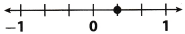(B)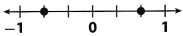(C)(D)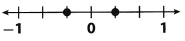(D)Explaination:
The opposite of a number is the number on the other side of the number line, and the same distance from 0.
Let’s draw our number line which will include $$\frac{1}{3}$$ and its opposite, –$$\frac{1}{3}$$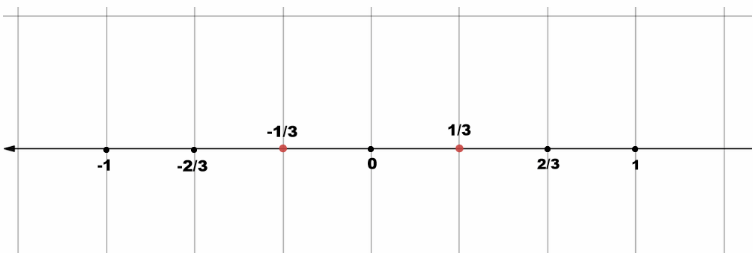Note that we used 1/3 instead of $$\frac{1}{3}$$.

Question 10.
Which of the following shows the numbers in order from least to greatest?
(A) – $$\frac{2}{3}$$, –$$\frac{3}{4}$$, 0.7, 0
(B) 0.7, 0, – $$\frac{2}{3}$$, – $$\frac{3}{4}$$,
(C) – $$\frac{2}{3}$$, – $$\frac{3}{4}$$, 0, 0.7
(D) – $$\frac{3}{4}$$, – $$\frac{2}{3}$$, 0, 0.7
(D) – $$\frac{3}{4}$$, – $$\frac{2}{3}$$, 0, 0.7

Explaination:
Given numbers are: – $$\frac{2}{3}$$, –$$\frac{3}{4}$$, 0.7, 0
Let’s order them from least to greatest!
The first thing is to transform them into equal form(decimals)!
– $$\frac{2}{3}$$ = – 0.$$\dot{6}$$
– $$\frac{3}{4}$$ = – 0.75
Now we can say that:
Number – 0.75 in less than – 0.$$\dot{6}$$ which in less than 0 which is less than 0.7
Therefore, we can write the an:
– 0.75 < – 0.$$\dot{6}$$ < 0 < 0.7
Now, we can return them into given forms and write them in the right order!
– $$\frac{3}{4}$$, – $$\frac{2}{3}$$, 0, 0.7

Division: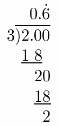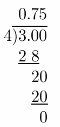Question 11.
Which number line shows an integer and its opposite?
(A)(B)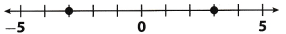(C)(D)(B)Explaination:
Here, we could draw all 4 of given number tines, and conclude which one is correct!
However, there is no purpose to do so.
Let’s see what are we actually looking for, when finding our answer.
We want to find the one, which shows an integer and its opposite.
As by definition, the opposite of a number is the number on the other side of 0 number line, and the same distance from 0
So, we should have same distance from 0 on both sides!
There is only 1 graph that satisfies our terms.
It is:Gridded Response

Question 12.
Which is the greatest out of $$\frac{1}{3}$$, – 1.2, 0.45, and – $$\frac{4}{5}$$?Given numbers are: $$\frac{1}{3}$$, – $$\frac{4}{5}$$, – 1.2. 0.45
Let’s order them from least to greatest, and then we will know our final solution!
First thing is to transform them into equal form(decimals)!
$$\frac{1}{3}$$ = 0.3
– $$\frac{4}{5}$$ = – 0.8
Now, we can say that:
Number – 1.2 is less than – 0.8 which is less than 0.$$\dot{3}$$ which is less than 0.45.
Therefore, we can write them as:
– 1.2 < – 0.8 < 0.$$\dot{3}$$ < 0.45
Now, if we take a look on previous expression, we can see that 0.45 is the greatest number among given ones!

Division: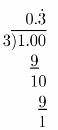Question 13.
As part of a research team, Ryanne climbed into a cavern to an elevation of – 117.6 feet. What is the absolute value of Ryanne’s elevation, in feet?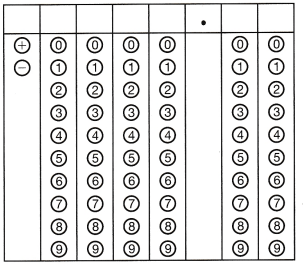In this task, we want to find the absolute value of given elevation!
Absolute value describes the distance of a number on the number line from 0 without considering which direction from zero the number lies.
The absolute value of a number is never negative.
|- 117.6| = 117.6 feet
= 117.6

Hot Tip: Correct answers in gridded problems can be positive or negative. Enter the negative sign in the first column when it is appropriate. Check your work!

Question 14.
Melvin has a certain number of files on his computer. The opposite of this number is – 653. How many files are on Melvin’s computer?